This book is archived and will be removed July 6, 2022. Please use the updated version.

Alternating-Current Circuits

# 104 Resonance in an AC Circuit

### Learning Objectives

By the end of the section, you will be able to:

• Determine the peak ac resonant angular frequency for a RLC circuit
• Explain the width of the average power versus angular frequency curve and its significance using terms like bandwidth and quality factor

In the RLC series circuit of (Figure), the current amplitude is, from (Figure),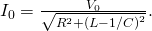If we can vary the frequency of the ac generator while keeping the amplitude of its output voltage constant, then the current changes accordingly. A plot of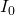versusis shown in (Figure).

At an RLC circuit’s resonant frequency,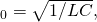the current amplitude is at its maximum value.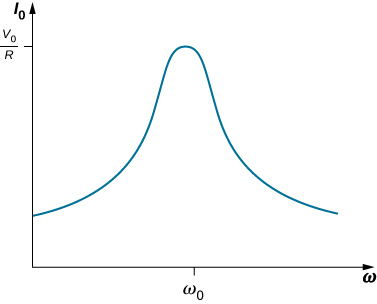In Oscillations, we encountered a similar graph where the amplitude of a damped harmonic oscillator was plotted against the angular frequency of a sinusoidal driving force (see Forced Oscillations). This similarity is more than just a coincidence, as shown earlier by the application of Kirchhoff’s loop rule to the circuit of (Figure). This yields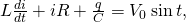or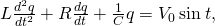where we substituted dq(t)/dt for i(t). A comparison of (Figure) and, from Oscillations, Damped Oscillations for damped harmonic motion clearly demonstrates that the driven RLC series circuit is the electrical analog of the driven damped harmonic oscillator.

The resonant frequency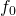of the RLC circuit is the frequency at which the amplitude of the current is a maximum and the circuit would oscillate if not driven by a voltage source. By inspection, this corresponds to the angular frequency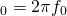at which the impedance Z in (Figure) is a minimum, or when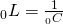and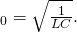This is the resonant angular frequency of the circuit. Substitutinginto (Figure), (Figure), and (Figure), we find that at resonance,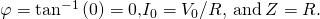Therefore, at resonance, an RLC circuit is purely resistive, with the applied emf and current in phase.

What happens to the power at resonance? (Figure) tells us how the average power transferred from an ac generator to the RLC combination varies with frequency. In addition,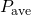reaches a maximum when Z, which depends on the frequency, is a minimum, that is, when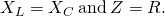Thus, at resonance, the average power output of the source in an RLC series circuit is a maximum. From (Figure), this maximum is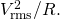(Figure) is a typical plot ofversusin the region of maximum power output. The bandwidthof the resonance peak is defined as the range of angular frequenciesover which the average poweris greater than one-half the maximum value of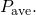The sharpness of the peak is described by a dimensionless quantity known as the quality factor Q of the circuit. By definition,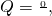whereis the resonant angular frequency. A high Q indicates a sharp resonance peak. We can give Q in terms of the circuit parameters as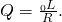Like the current, the average power transferred from an ac generator to an RLC circuit peaks at the resonant frequency.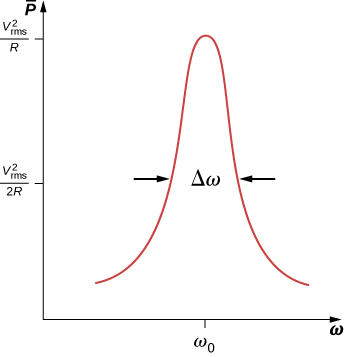Resonant circuits are commonly used to pass or reject selected frequency ranges. This is done by adjusting the value of one of the elements and hence “tuning” the circuit to a particular resonant frequency. For example, in radios, the receiver is tuned to the desired station by adjusting the resonant frequency of its circuitry to match the frequency of the station. If the tuning circuit has a high Q, it will have a small bandwidth, so signals from other stations at frequencies even slightly different from the resonant frequency encounter a high impedance and are not passed by the circuit. Cell phones work in a similar fashion, communicating with signals of around 1 GHz that are tuned by an inductor-capacitor circuit. One of the most common applications of capacitors is their use in ac-timing circuits, based on attaining a resonant frequency. A metal detector also uses a shift in resonance frequency in detecting metals ((Figure)).

When a metal detector comes near a piece of metal, the self-inductance of one of its coils changes. This causes a shift in the resonant frequency of a circuit containing the coil. That shift is detected by the circuitry and transmitted to the diver by means of the headphones. (credit: modification of work by Eric Lippmann, U.S. Navy)Resonance in an RLC Series Circuit (a) What is the resonant frequency of the circuit of (Figure)? (b) If the ac generator is set to this frequency without changing the amplitude of the output voltage, what is the amplitude of the current?

Strategy The resonant frequency for a RLC circuit is calculated from (Figure), which comes from a balance between the reactances of the capacitor and the inductor. Since the circuit is at resonance, the impedance is equal to the resistor. Then, the peak current is calculated by the voltage divided by the resistance.

Solution

1. The resonant frequency is found from (Figure):
*** QuickLaTeX cannot compile formula:
\begin{array}{}\\ \\ \hfill {f}_{0}& =\frac{1}{2\pi }\sqrt{\frac{1}{LC}}=\frac{1}{2\pi }\phantom{\rule{0.2em}{0ex}}\sqrt{\frac{1}{\left(3.00\phantom{\rule{0.2em}{0ex}}×\phantom{\rule{0.2em}{0ex}}{10}^{-3}\phantom{\rule{0.2em}{0ex}}\text{H}\right)\left(8.00\phantom{\rule{0.2em}{0ex}}×\phantom{\rule{0.2em}{0ex}}{10}^{-4}\phantom{\rule{0.2em}{0ex}}\text{F}\right)}}\hfill \\ & =1.03\phantom{\rule{0.2em}{0ex}}×\phantom{\rule{0.2em}{0ex}}{10}^{2}\phantom{\rule{0.2em}{0ex}}\text{Hz}\text{.}\hfill \end{array}

*** Error message:
Missing # inserted in alignment preamble.
leading text: $\begin{array}{} Missing$ inserted.
leading text: $\begin{array}{}\\ \\ \hfill {f}_ Missing$ inserted.
leading text: \begin{array}{}\\ \\ \hfill {f}_{0}& Extra alignment tab has been changed to \cr. leading text:\begin{array}{}\\ \\ \hfill {f}_{0}&
Missing $inserted. leading text: ...ay}{}\\ \\ \hfill {f}_{0}& =\frac{1}{2\pi } Extra }, or forgotten$.
leading text: ...ay}{}\\ \\ \hfill {f}_{0}& =\frac{1}{2\pi }
Package inputenc Error: Unicode character × (U+00D7)
Package inputenc Error: Unicode character × (U+00D7)
Missing } inserted.


2. At resonance, the impedance of the circuit is purely resistive, and the current amplitude is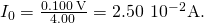Significance If the circuit were not set to the resonant frequency, we would need the impedance of the entire circuit to calculate the current.

Power Transfer in an RLC Series Circuit at Resonance (a) What is the resonant angular frequency of an RLC circuit with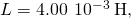and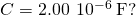(b) If an ac source of constant amplitude 4.00 V is set to this frequency, what is the average power transferred to the circuit? (c) Determine Q and the bandwidth of this circuit.

Strategy The resonant angular frequency is calculated from (Figure). The average power is calculated from the rms voltage and the resistance in the circuit. The quality factor is calculated from (Figure) and by knowing the resonant frequency. The bandwidth is calculated from (Figure) and by knowing the quality factor.

Solution

1. The resonant angular frequency is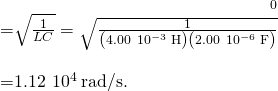2. At this frequency, the average power transferred to the circuit is a maximum. It is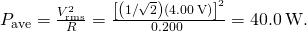3. The quality factor of the circuit is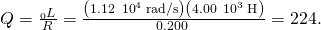We then find for the bandwidth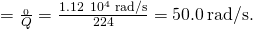Significance If a narrower bandwidth is desired, a lower resistance or higher inductance would help. However, a lower resistance increases the power transferred to the circuit, which may not be desirable, depending on the maximum power that could possibly be transferred.

Check Your Understanding In the circuit of (Figure),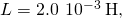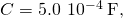and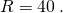(a) What is the resonant frequency? (b) What is the impedance of the circuit at resonance? (c) If the voltage amplitude is 10 V, what is i(t) at resonance? (d) The frequency of the AC generator is now changed to 200 Hz. Calculate the phase difference between the current and the emf of the generator.

a. 160 Hz; b.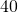; c.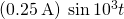; d. 0.023 rad

Check Your Understanding What happens to the resonant frequency of an RLC series circuit when the following quantities are increased by a factor of 4: (a) the capacitance, (b) the self-inductance, and (c) the resistance?

a. halved; b. halved; c. same

Check Your Understanding The resonant angular frequency of an RLC series circuit is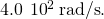An ac source operating at this frequency transfers an average power of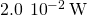to the circuit. The resistance of the circuit is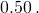Write an expression for the emf of the source.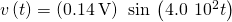### Summary

• At the resonant frequency, inductive reactance equals capacitive reactance.
• The average power versus angular frequency plot for a RLC circuit has a peak located at the resonant frequency; the sharpness or width of the peak is known as the bandwidth.
• The bandwidth is related to a dimensionless quantity called the quality factor. A high quality factor value is a sharp or narrow peak.

### Problems

(a) Calculate the resonant angular frequency of an RLC series circuit for which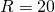,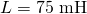, and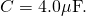(b) If R is changed to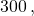what happens to the resonant angular frequency?

The resonant frequency of an RLC series circuit is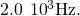If the self-inductance in the circuit is 5.0 mH, what is the capacitance in the circuit?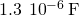(a) What is the resonant frequency of an RLC series circuit with,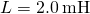, and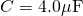? (b) What is the impedance of the circuit at resonance?

For an RLC series circuit,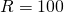,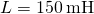, and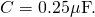(a) If an ac source of variable frequency is connected to the circuit, at what frequency is maximum power dissipated in the resistor? (b) What is the quality factor of the circuit?

a. 820 Hz; b. 7.8

An ac source of voltage amplitude 100 V and variable frequency f drives an RLC series circuit with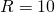,, and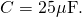(a) Plot the current through the resistor as a function of the frequency f. (b) Use the plot to determine the resonant frequency of the circuit.

(a) What is the resonant frequency of a resistor, capacitor, and inductor connected in series if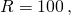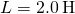, and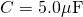? (b) If this combination is connected to a 100-V source operating at the resonant frequency, what is the power output of the source? (c) What is the Q of the circuit? (d) What is the bandwidth of the circuit?

a. 50 Hz; b. 50 W; c. 6.32; d. 50 rad/s

Suppose a coil has a self-inductance of 20.0 H and a resistance of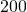. What (a) capacitance and (b) resistance must be connected in series with the coil to produce a circuit that has a resonant frequency of 100 Hz and a Q of 10?

An ac generator is connected to a device whose internal circuits are not known. We only know current and voltage outside the device, as shown below. Based on the information given, what can you infer about the electrical nature of the device and its power usage?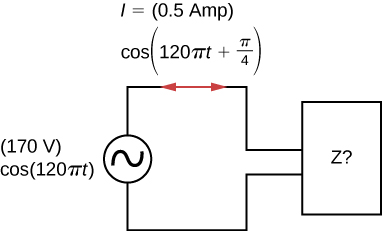The reactance of the capacitor is larger than the reactance of the inductor because the current leads the voltage. The power usage is 30 W.

### Glossary

bandwidth
range of angular frequencies over which the average power is greater than one-half the maximum value of the average power
quality factor
dimensionless quantity that describes the sharpness of the peak of the bandwidth; a high quality factor is a sharp or narrow resonance peak
resonant frequency
frequency at which the amplitude of the current is a maximum and the circuit would oscillate if not driven by a voltage source